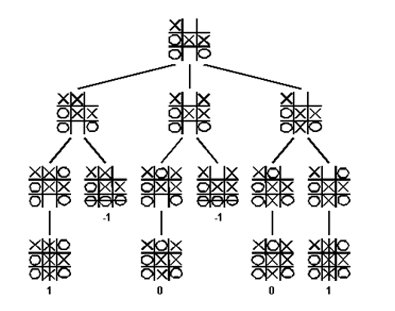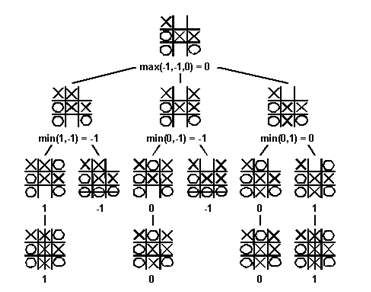2 views

I'm having a huge block trying to understand "trees" while making a Tic-Tac-Toe bot. I understand the concept, but I can't figure out to implement them.

Can someone show me an example of how a tree should be generated for such a case? Or a good tutorial on generating trees? I guess the hard part is generating partial trees. I know how to implement generating a whole tree, but not parts of it.

by (108k points)

We consider games with two players in which one person's gains are the result of another person's losses (so-called zero-sum games). The minimax algorithm is a specialized search algorithm that returns the optimal sequence of moves for a player in a zero-sum game. In the game tree that results from the algorithm, each level represents a move by either of two players, say A- and B-player. Below is a game tree for the tic-tac-toe game:The minimax algorithm explores the entire game tree using a depth-first search. At each node in the tree where A-player has to move, A-player would like to play the move that maximizes the payoff. Thus, A-player will assign the maximum score amongst the children to the node where Max makes a move. Similarly, B-player will minimize the payoff to A-player. The maximum and minimum scores are taken at alternating levels of the tree since A and B alternate turns.The minimax algorithm computes the minimax decision for the leaves of the game tree and then backs up through the tree to give the final value to the current state.

Here is the code you can implement:

https://www.geeksforgeeks.org/minimax-algorithm-in-game-theory-set-3-tic-tac-toe-ai-finding-optimal-move/

If you are looking to learn more about Artificial Intelligence then you visit Artificial Intelligence Tutorial. Also, if you are appearing for job profiles of AI Engineer or AI Expert then you can prepare for the interviews on Artificial Intelligence Interview Questions.